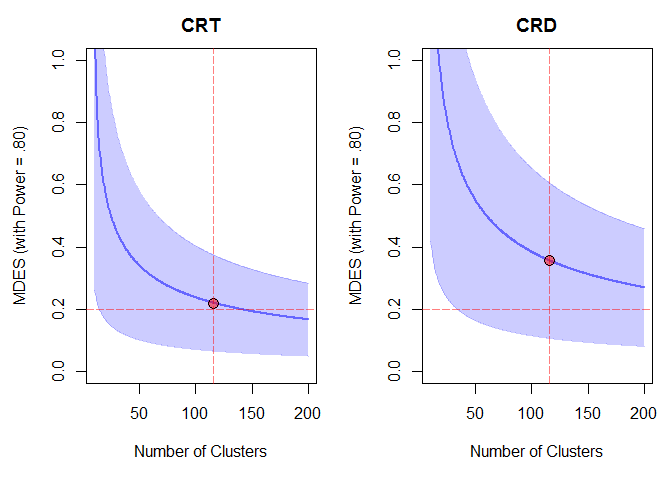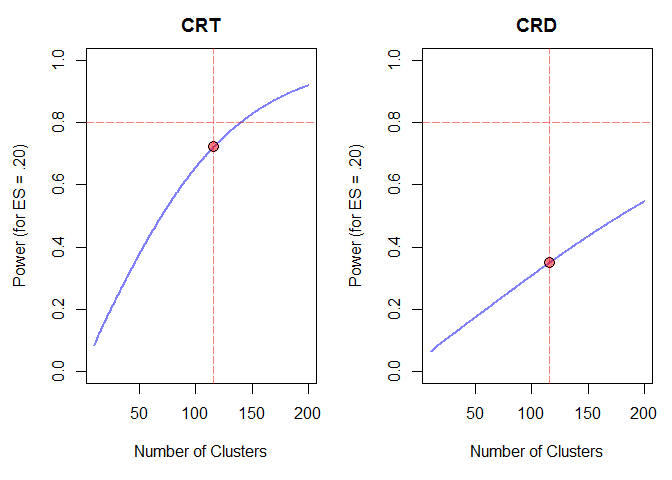# Bound Constrained Optimal Design of Multilevel Regression Discontinuity Designs and Randomized Controlled Trials

#### 2019-05-09

To install and load the package:

install.packages("cosa")
library(cosa)

The following examples demonstrate how to perform bound constrained optimal sample allocation (BCOSA) for a two-level cluster randomized trial (CRT) and for the corresponding cluster-level regression discontinuity (CRD) design under three primary constraints. Note: NULL arguments are provided for clarity, otherwise they do not have to be explicit.

## Primary Constraint on the Total Cost

# CRT('rhots = 0')
# cost constrained - optimize p and n2
crt <- cosa.crd2r2(rhots = 0,
constrain = "cost", cost = 12500,
cn1 = c(5, 2), cn2 = c(50, 20),
es = .20,  power = .80, rho2 = .20,
g2 = 5, r21 = .20, r22 = .30,
p = NULL, n1 = 24,  n2 = NULL)
##
## Results are equivalent to corresponding random assignment designs
## Solution converged with LBFGS
##
## Rounded solution:
## ---------------------------------------------------
##  [n1]  n2     p [cost] mdes 95%lcl 95%ucl power
##    24 116 0.388  12478 0.22  0.066  0.374 0.722
## ---------------------------------------------------
## Per unit marginal costs:
##  Level 1 treatment: 5
##  Level 1 control: 2
##  Level 2 treatment: 50
##  Level 2 control: 20
## ---------------------------------------------------
## MDES = 0.22 (with power = 80)
## power = 0.722 (for ES = 0.2)
## ---------------------------------------------------
## []: point constrained (fixed)
## <<: bound constrained
# CRD
# cost constrained - optimize n2
crd <- cosa.crd2r2(constrain = "cost", cost = 12500,
cn1 = c(5, 2), cn2 = c(50, 20),
es = .20,  power = .80, rho2 = .20,
g2 = 5, r21 = .20, r22 = .30,
p = .387, n1 = 24,  n2 = NULL)
## Solution converged with LBFGS
##
## Rounded solution:
## ---------------------------------------------------
##  [n1]  n2   [p] [cost]  mdes 95%lcl 95%ucl power
##    24 116 0.388  12478 0.356  0.106  0.605 0.351
## ---------------------------------------------------
## Per unit marginal costs:
##  Level 1 treatment: 5
##  Level 1 control: 2
##  Level 2 treatment: 50
##  Level 2 control: 20
## ---------------------------------------------------
## MDES = 0.356 (with power = 80)
## power = 0.351 (for ES = 0.2)
## ---------------------------------------------------
## []: point constrained (fixed)
## <<: bound constrained
# example plots
par(mfrow = c(1, 2), mai = c(1, .9, .5, .2))
# compare minimum detectable effect size and 95% CI
plot(crt, ypar = "mdes", xpar = "n2",
ylim = c(0, 1), xlim = c(10, 200),
ylab = "MDES (with Power = .80)", xlab = "Number of Clusters",
main = "CRT", locate = TRUE)
plot(crd, ypar = "mdes", xpar = "n2",
ylim = c(0, 1), xlim = c(10, 200),
ylab = "MDES (with Power = .80)", xlab = "Number of Clusters",
main = "CRD", locate = TRUE)# compare statistical power
plot(crt, ypar = "power", xpar = "n2",
ylim = c(0, 1), xlim = c(10, 200),
ylab = "Power (for ES = .20)", xlab = "Number of Clusters",
main = "CRT", locate = TRUE)
plot(crd, ypar = "power", xpar = "n2",
ylim = c(0, 1), xlim = c(10, 200),
ylab = "Power (for ES = .20)", xlab = "Number of Clusters",
main = "CRD", locate = TRUE)As seen from the MDES and power plots, a little less than 150 clusters are needed to obtain the benchmark power rate of 80% for the CRT (more than 200 clusters for the CRD). Precise number of clusters can be found via placing the primary constraint on either the effect size or power rate.

## Primary Constraint on the Effect Size

# CRT('rhots = 0')
# cost constrained - optimize p and n2
cosa.crd2r2(rhots = 0,
constrain = "es", es = .20,
cn1 = c(5, 2), cn2 = c(50, 20),
power = .80, rho2 = .20,
g2 = 5, r21 = .20, r22 = .30,
p = NULL, n1 = 24,  n2 = NULL)
##
## Results are equivalent to corresponding random assignment designs
## Solution converged with SLSQP
##
## Rounded solution:
## ---------------------------------------------------
##  [n1]  n2     p  cost [mdes] 95%lcl 95%ucl power
##    24 140 0.386 15028    0.2   0.06   0.34   0.8
## ---------------------------------------------------
## Per unit marginal costs:
##  Level 1 treatment: 5
##  Level 1 control: 2
##  Level 2 treatment: 50
##  Level 2 control: 20
## ---------------------------------------------------
## MDES = 0.2 (with power = 80)
## power = 0.8 (for ES = 0.2)
## ---------------------------------------------------
## []: point constrained (fixed)
## <<: bound constrained
# CRD
# cost constrained - optimize n2
cosa.crd2r2(constrain = "es", es = .20,
power = .80, rho2 = .20,
cn1 = c(5, 2), cn2 = c(50, 20),
g2 = 5, r21 = .20, r22 = .30,
p = .386, n1 = 24,  n2 = NULL)
## Solution converged with SLSQP
##
## Rounded solution:
## ---------------------------------------------------
##  [n1]  n2   [p]  cost [mdes] 95%lcl 95%ucl power
##    24 363 0.386 38964    0.2   0.06   0.34   0.8
## ---------------------------------------------------
## Per unit marginal costs:
##  Level 1 treatment: 5
##  Level 1 control: 2
##  Level 2 treatment: 50
##  Level 2 control: 20
## ---------------------------------------------------
## MDES = 0.2 (with power = 80)
## power = 0.8 (for ES = 0.2)
## ---------------------------------------------------
## []: point constrained (fixed)
## <<: bound constrained

## Primary Constraint on the Power Rate

# CRT('rhots = 0')
# cost constrained - optimize p and n2
cosa.crd2r2(rhots = 0,
constrain = "power", power = .80,
cn1 = c(5, 2), cn2 = c(50, 20),
es = .20, rho2 = .20,
g2 = 5, r21 = .20, r22 = .30,
p = NULL, n1 = 24,  n2 = NULL)
##
## Results are equivalent to corresponding random assignment designs
## Solution converged with SLSQP
##
## Rounded solution:
## ---------------------------------------------------
##  [n1]  n2     p  cost mdes 95%lcl 95%ucl [power]
##    24 140 0.386 15028  0.2   0.06   0.34     0.8
## ---------------------------------------------------
## Per unit marginal costs:
##  Level 1 treatment: 5
##  Level 1 control: 2
##  Level 2 treatment: 50
##  Level 2 control: 20
## ---------------------------------------------------
## MDES = 0.2 (with power = 80)
## power = 0.8 (for ES = 0.2)
## ---------------------------------------------------
## []: point constrained (fixed)
## <<: bound constrained
# the cost reduction for using an unbalanced design might be trivial
# fix p = .50 to obtain the cost under the balanced design for comparison
cosa.crd2r2(rhots = 0,
constrain = "power", power = .80,
cn1 = c(5, 2), cn2 = c(50, 20),
es = .20, rho2 = .20,
g2 = 5, r21 = .20, r22 = .30,
p = .50, n1 = 24,  n2 = NULL)
##
## Results are equivalent to corresponding random assignment designs
## Solution converged with SLSQP
##
## Rounded solution:
## ---------------------------------------------------
##  [n1]  n2   [p]  cost mdes 95%lcl 95%ucl [power]
##    24 133 0.496 15776  0.2   0.06   0.34     0.8
## ---------------------------------------------------
## Per unit marginal costs:
##  Level 1 treatment: 5
##  Level 1 control: 2
##  Level 2 treatment: 50
##  Level 2 control: 20
## ---------------------------------------------------
## MDES = 0.2 (with power = 80)
## power = 0.8 (for ES = 0.2)
## ---------------------------------------------------
## []: point constrained (fixed)
## <<: bound constrained
# CRD
# cost constrained - optimize n2
cosa.crd2r2(constrain = "power", power = .80,
cn1 = c(5, 2), cn2 = c(50, 20),
es = .20, rho2 = .20,
g2 = 5, r21 = .20, r22 = .30,
p = .386, n1 = 24,  n2 = NULL)
## Solution converged with SLSQP
##
## Rounded solution:
## ---------------------------------------------------
##  [n1]  n2   [p]  cost mdes 95%lcl 95%ucl [power]
##    24 363 0.386 38964  0.2   0.06   0.34     0.8
## ---------------------------------------------------
## Per unit marginal costs:
##  Level 1 treatment: 5
##  Level 1 control: 2
##  Level 2 treatment: 50
##  Level 2 control: 20
## ---------------------------------------------------
## MDES = 0.2 (with power = 80)
## power = 0.8 (for ES = 0.2)
## ---------------------------------------------------
## []: point constrained (fixed)
## <<: bound constrained

–o–